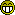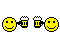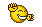###Author Topic: Help with Yabsic converting  (Read 1559 times)

0 Members and 1 Guest are viewing this topic.

####emook##### Help with Yabsic converting
« on: January 08, 2014 »
I am trying to convert some copper bars by Jim to PB (source is here : http://www.dbfinteractive.com/forum/index.php/topic,752.0.html) , however, Yabasic has a bitwise compare and I cannot work out the PB alternate.

Code: [Select]
`; 'twisty fatty shrinky barry thing, with copper bars; 'by Jim 18/10/2006sw=640sh=480hsw=sw/2;open window sw,shOpenWindow(0,100,100,640,480,"") : InitSprite()OpenWindowedScreen(WindowID(0),0,0,640,480);';twisty barry thing stuffascale.f=3.14/180w.f=100inc.f=0iinc.f=0.01Dim c(4,3)c(0,0)=255:c(0,1)=0:c(0,2)=0c(1,0)=0:c(1,1)=0:c(1,2)=255c(2,0)=0:c(2,1)=255:c(2,2)=0c(3,0)=255:c(3,1)=255:c(3,2)=0c(4,0)=c(0,0):c(4,1)=c(0,1):c(4,2)=c(0,2);'copper bar stuffDim cuy.f(3)Dim cui.f(3)Dim RGBc.f(3)cuy(0)=0:cuy(1)=0:cuy(2)=0cui(0)=0.005:cui(1)=0.007:cui(2)=0.012cuh=80Repeat ;setdispbuf d=1-d ;setdrawbuf d ClearScreen(0)StartDrawing(ScreenOutput()) For y=0 To sh-1 RGBc(0)=0 RGBc(1)=0 RGBc(2)=0 For c=0 To 2     If y>cuy(c) And y<cuy(c)+cuh RGBc(c)=(cuh/2-Abs(y-cuy(c)-(cuh/2)))*(255/cuh) EndIf cuy(c)=cuy(c)+cui(c) If cuy(c)>=sh-cuh Or cuy(c)<=0 cui(c)=-cui(c) EndIf Next If RGBc(0)<>0 Or RGBc(1)<>0 Or RGBc(2)<>0 ;  Debug "LineW" ;setrgb 1,RGBc(0),RGBc(1),RGBc(2) Line(0,y,sw,y,RGB(RGBc(0),RGBc(1),RGBc(2))) ;Line(0,y,sw,y,#Red) EndIf Next a=0 inc=inc+iinc For y=0 To sh-100*Abs(inc) x1 = w * (7+Abs(inc))/7 * Sin(a*ascale) x2 = w * (7+Abs(inc))/7 * Cos(a*ascale) x1=Abs(x1):x2=Abs(x2) q=Int(a/90) If q & 1<>0;   If q <>0  t = x1:x1 = x2:x2 = t  EndIf ow.f = hsw-((x1+x2)/2) ;setrgb 1,c(q+1,0),c(q+1,1),c(q+1,2) Line(ow,y,ow+x1,y,RGB(c(q+1,0),c(q+1,1),c(q+1,2))) ;Line(ow,y,ow+x1,y,#Red) ;setrgb 1,c(q,0),c(q,1),c(q,2) Line(ow+x1,y,ow+x1+x2,y,RGB(c(q,0),c(q,1),c(q,2))) ;Line(ow+x1,y,ow+x1+x2,y,#Red) a=a+inc If a<0 : a=a+360: EndIf If a>=360 : a=a-360: EndIf Next If inc>2 Or inc<-2 : iinc=-iinc : EndIf StopDrawing() FlipBuffers()Until quit=1; ; sub copperbars(); For y=0 To sh-1; RGB(0)=0; RGB(1)=0; RGB(2)=0; For c=0 To 2; If y>cuy(c) And y<cuy(c)+cuh then; RGB(c)=(cuh/2-Abs(y-cuy(c)-(cuh/2)))*(255/cuh); fi; cuy(c)=cuy(c)+cui(c); If cuy(c)>=sh-cuh Or cuy(c)<=0 then; cui(c)=-cui(c); fi; Next; If RGB(0)<>0 Or RGB(1)<>0 Or RGB(2)<>0 then; setrgb 1,RGB(0),RGB(1),RGB(2); line 0,y,sw,y; fi; Next; End sub`
----

####ninogenio

• Pentium
•• Posts: 1667
• Karma: 133##### Re: Help with Yabsic converting
« Reply #1 on: January 08, 2014 »
hi mate could you post the line your having trouble with? my old eyes are just not what they used too bei have never used purebasic but i have coded quite a bit in yabasic so should be able too help out.
« Last Edit: January 08, 2014 by ninogenio »

####emook##### Re: Help with Yabsic converting
« Reply #2 on: January 08, 2014 »
Thanks - Here is what it looks like in Purebasic - it doesnt seem quite right :I think it maybe this part of the original code :

Code: [Select]
`if and(q,1)<>0 thent = x1:x1 = x2:x2 = tfi`
Which I checked the yabasic reference which tells me :

Code: [Select]
`and()  the bitwise arithmetic and`
In PB we use & for a bitwise :

Code: [Select]
`&  - Bitwise AND. You should be familiar with binary numbers when using this operator. The result of this operator will be the value of the expression on the LHS anded with the value of the expression on the RHS, bit for bit. The value of each bit is set according to the table below. Additionally, if the result of the operator is not used and there is a variable on the LHS, then the result will be stored directly in that variable. This operator cannot be used with strings.`

I've attached an exe showing what happens.

« Last Edit: January 08, 2014 by emook »
----

####ninogenio

• Pentium
•• Posts: 1667
• Karma: 133##### Re: Help with Yabsic converting
« Reply #3 on: January 08, 2014 »
yay i just got it too work!

i just downloaded pb and it turns out you were really really close i just had too change your line functions too lineXY and also use floats in a few places where you were previously using int if you check here you will see the few small changes madeQuote
; 'twisty fatty shrinky barry thing, with copper bars
; 'by Jim 18/10/2006
sw=640
sh=480
hsw.f=sw/2
;open window sw,sh
OpenWindow(0,100,100,640,480,"") : InitSprite()
OpenWindowedScreen(WindowID(0),0,0,640,480)

;';twisty barry thing stuff
ascale.f=3.14/180
w.f=100
inc.f=0
iinc.f=0.01
Dim c(4,3)
c(0,0)=255:c(0,1)=0:c(0,2)=0
c(1,0)=0:c(1,1)=0:c(1,2)=255
c(2,0)=0:c(2,1)=255:c(2,2)=0
c(3,0)=255:c(3,1)=255:c(3,2)=0
c(4,0)=c(0,0):c(4,1)=c(0,1):c(4,2)=c(0,2)

;'copper bar stuff
Dim cuy.f(3)
Dim cui.f(3)
Dim RGBc.f(3)
cuy(0)=0:cuy(1)=0:cuy(2)=0
cui(0)=0.005:cui(1)=0.007:cui(2)=0.012
cuh=80

Repeat

ClearScreen(0)

StartDrawing(ScreenOutput())
For y=0 To sh-1
RGBc(0)=0
RGBc(1)=0
RGBc(2)=0
For c=0 To 2

If y>cuy(c) And y<cuy(c)+cuh
RGBc(c)=(cuh/2-Abs(y-cuy(c)-(cuh/2)))*(255/cuh)
EndIf

cuy(c)=cuy(c)+cui(c)

If cuy(c)>=sh-cuh Or cuy(c)<=0
cui(c)=-cui(c)
EndIf

Next

If RGBc(0)>0 Or RGBc(1)>0 Or RGBc(2)>0
;  Debug "LineW"
;setrgb 1,RGBc(0),RGBc(1),RGBc(2)
LineXY(0,y,sw,y,RGB(RGBc(0),RGBc(1),RGBc(2)))
;Line(0,y,sw,y,#Red)
EndIf

Next

a.f=0
inc.f=inc.f+iinc.f
For y=0 To sh-100*Abs(inc)
x1.f = w.f * (7.0+Abs(inc))/7.0 * Sin(a.f*ascale.f)
x2.f = w.f * (7.0+Abs(inc))/7.0 * Cos(a.f*ascale.f)
x1=Abs(x1):x2=Abs(x2)
q=Int(a/90)

If q & 1<>0
;         If q <>0
t = x1:x1 = x2:x2 = t
EndIf

ow.f = hsw.f-((x1+x2)/2)
;setrgb 1,c(q+1,0),c(q+1,1),c(q+1,2)

LineXY(ow,y,ow+x1,y,RGB(c(q+1,0),c(q+1,1),c(q+1,2)))
;Line(ow,y,ow+x1,y,#Red)

;setrgb 1,c(q,0),c(q,1),c(q,2)

LineXY(ow+x1,y,ow+x1+x2,y,RGB(c(q,0),c(q,1),c(q,2)))
;Line(ow+x1,y,ow+x1+x2,y,#Red)
a=a+inc

If a.f<0 : a.f=a.f+360: EndIf

If a.f>=360   : a.f=a.f-360: EndIf

Next

If inc.f>2 Or inc.f<-2 : iinc.f=-iinc.f : EndIf
StopDrawing()
FlipBuffers()
Until quit=1

####emook##### Re: Help with Yabsic converting
« Reply #4 on: January 08, 2014 »
Yay! Brilliant - that's what it should look like!

Thank you!

K++----

####Jim

• Founder Member
••